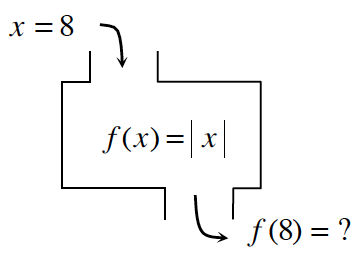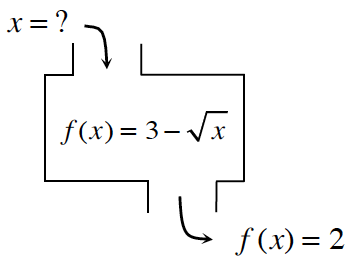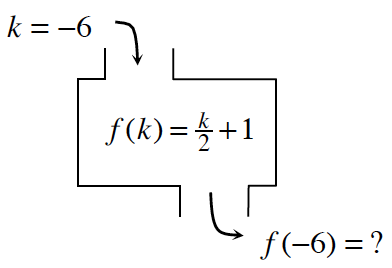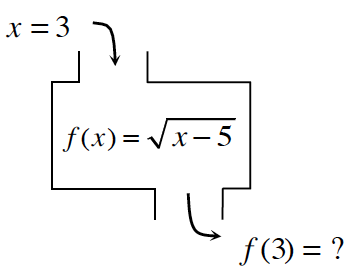Home > CCAA8 > Chapter cca1 > Lesson cca1.2.4 > Problem1-70

1-70.

Find the corresponding inputs or outputs for the following functions. If there is no solution, explain why not. Be careful: In some cases, there may be no solution or more than one possible solution. Homework Help ✎

1.• Substitute $x$ into the equation.

$f(8)=|8|$

1.Set up the equation.

$2=3 -\sqrt{x}$

Solve for $x$.

$x=1$

1.• Refer to part (a).

1.• Refer to part (a).

no solution